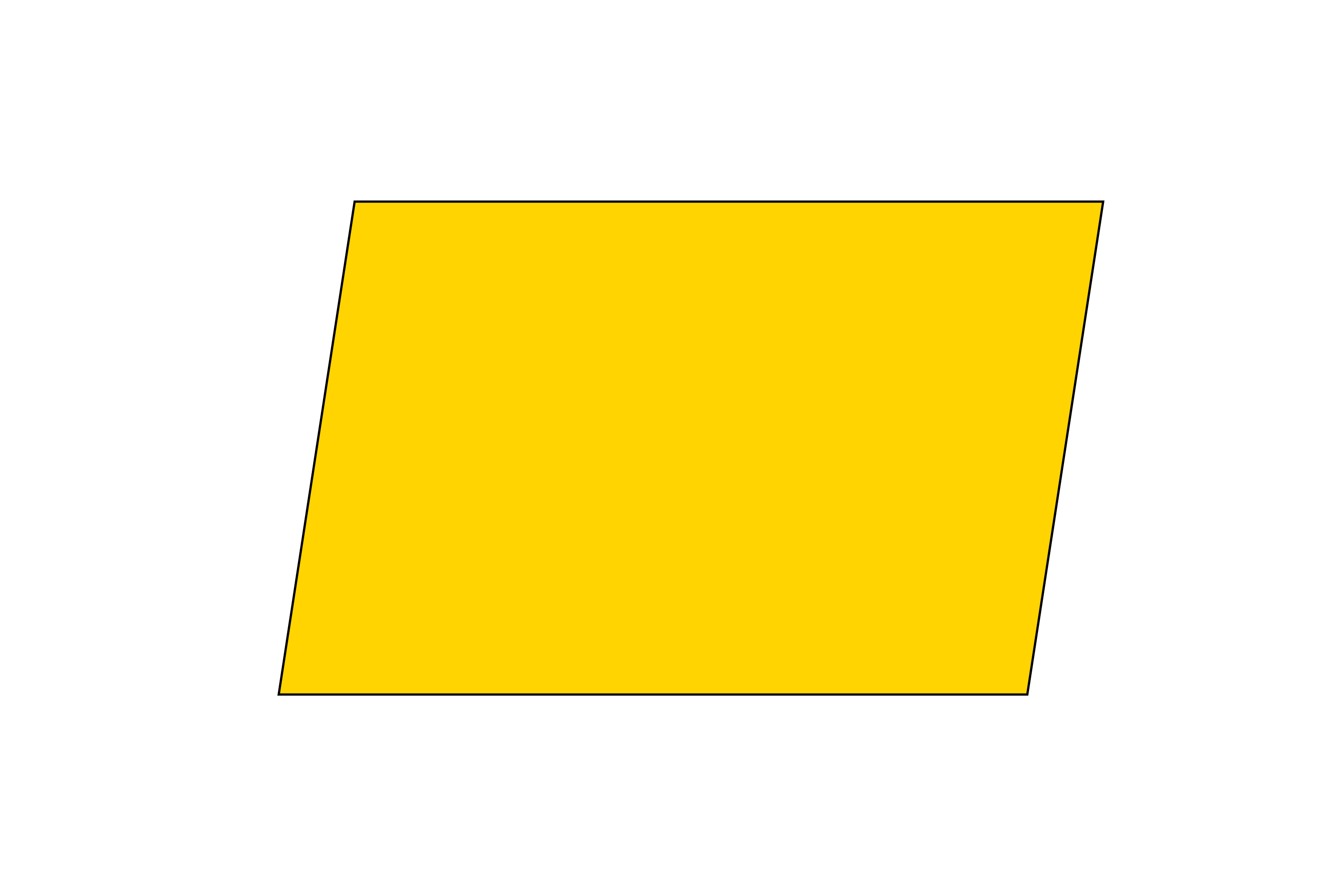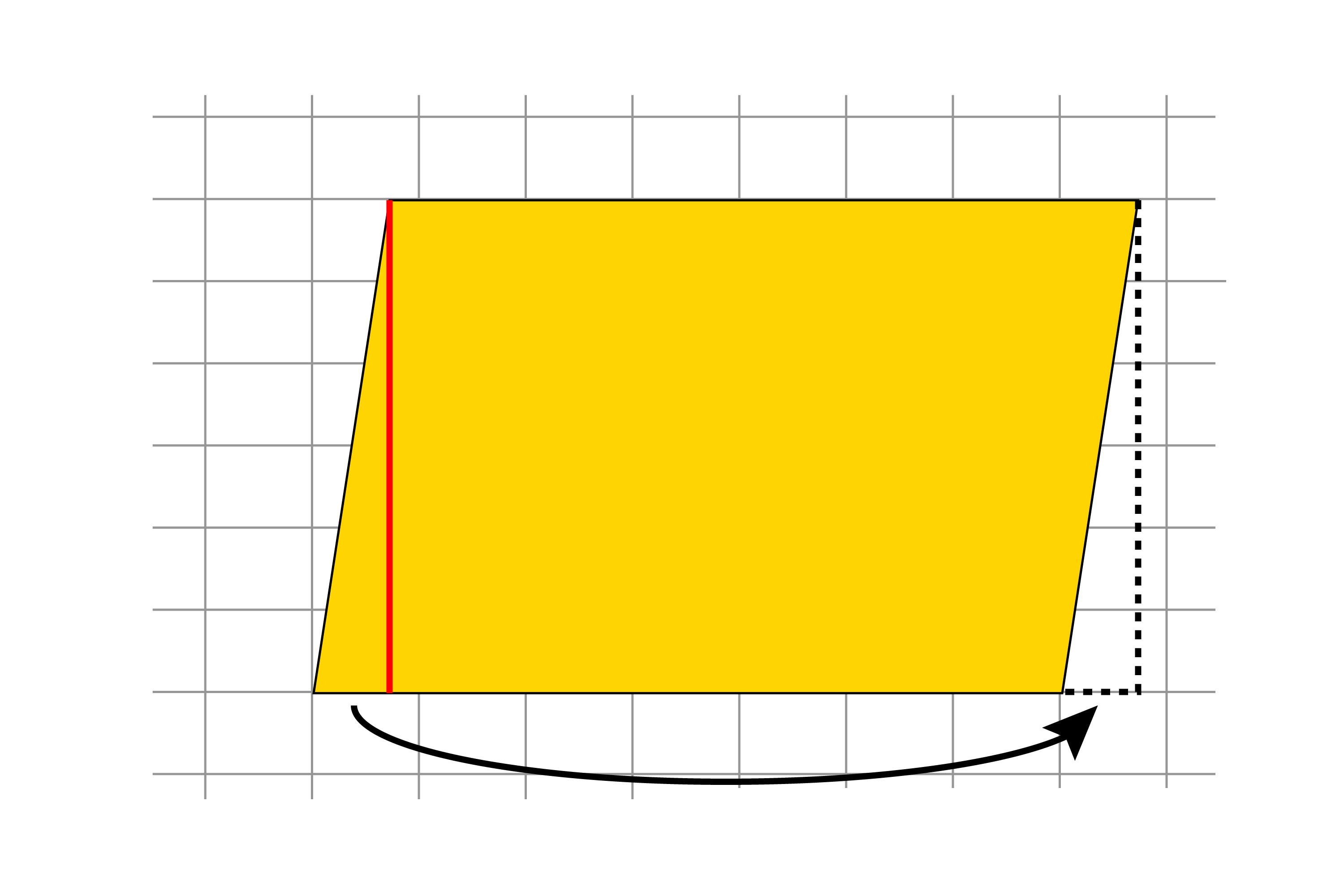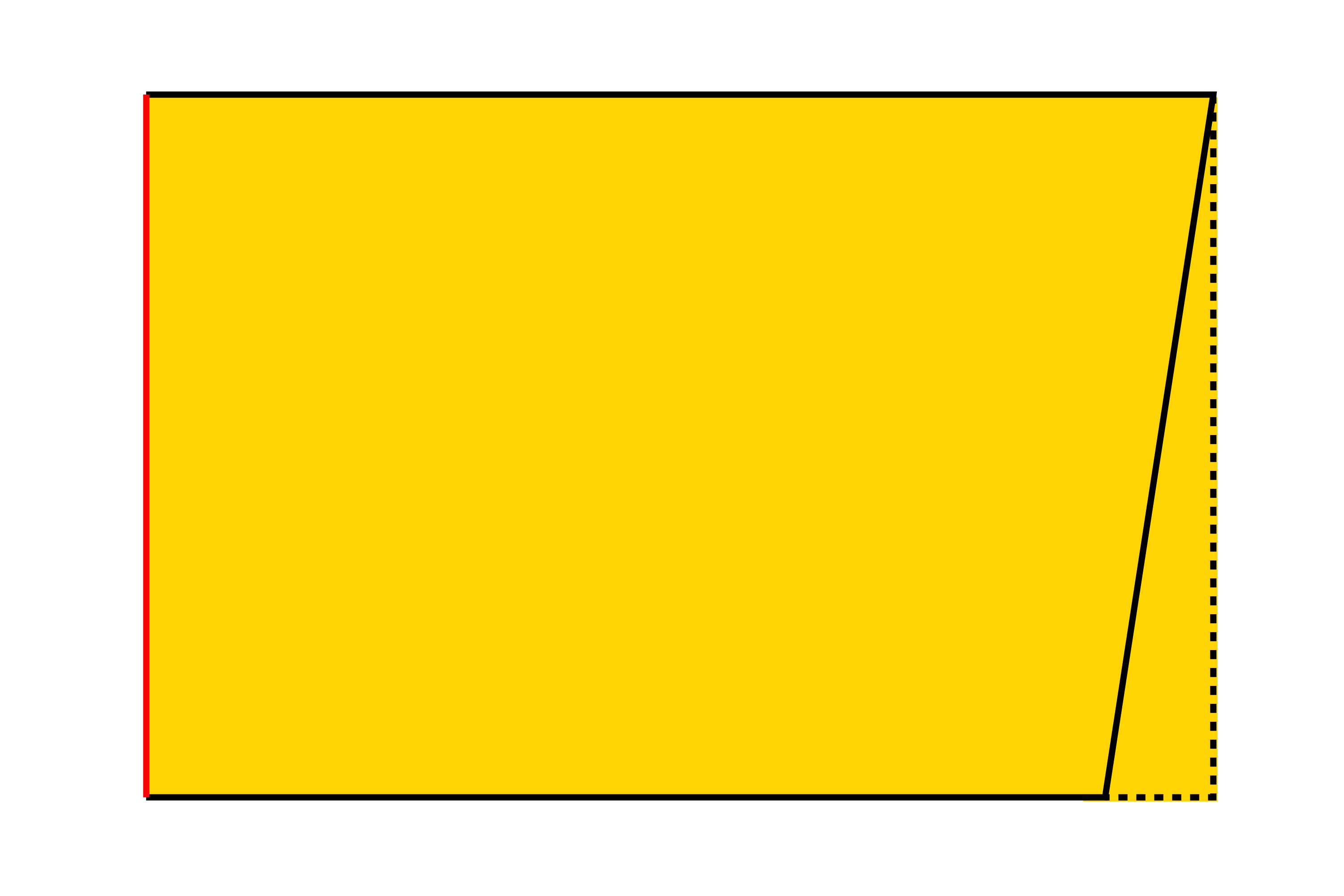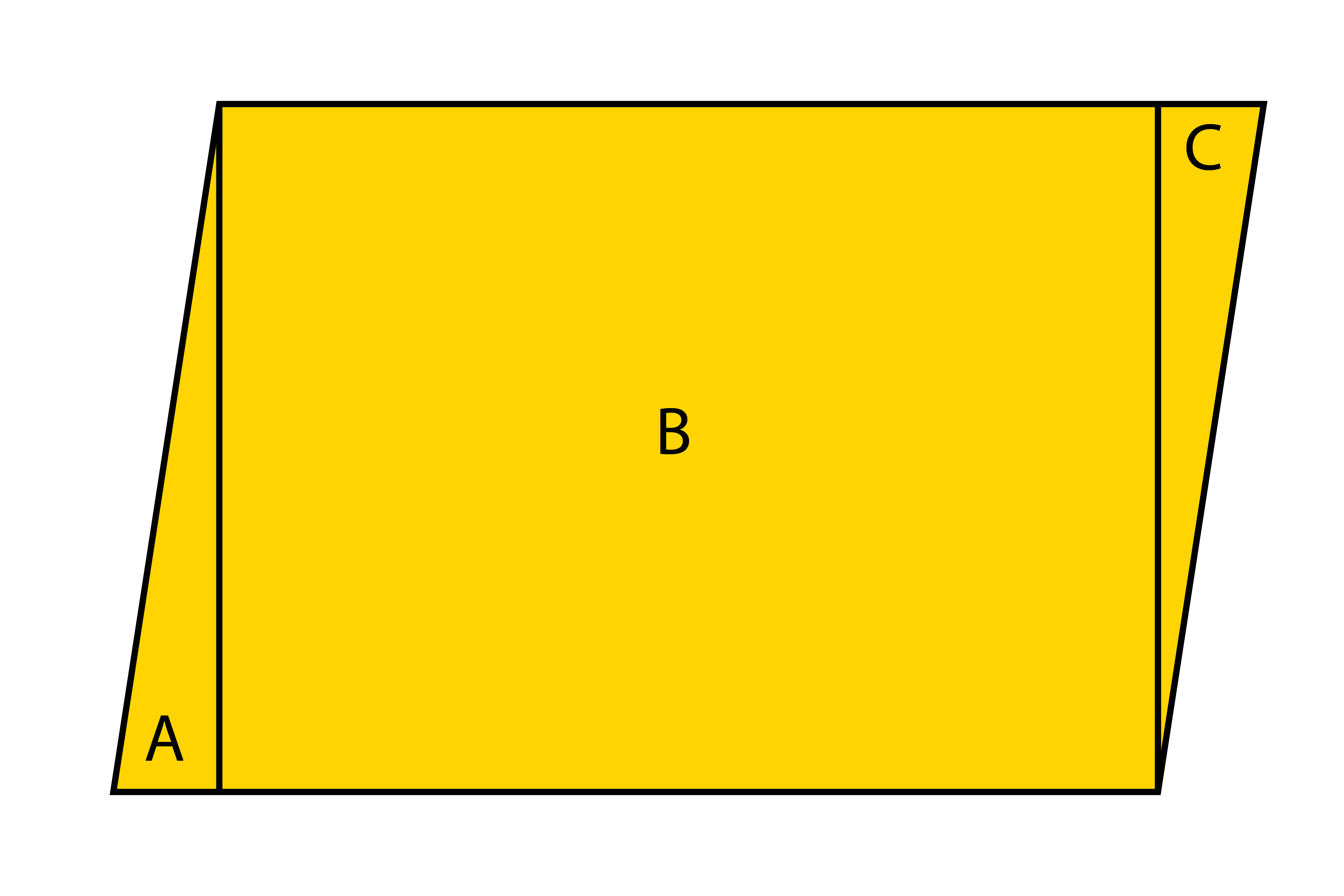# Area of a parallelogram

Again think in terms of a rectangle (see area of a triangle).RedrawThe left-hand triangle will fit exactly in the right-hand triangle.

Redraw again.So the area of a parallelogram is…

Base x Height (like a normal rectangle).

## AlternativelyIt could also be redrawn asThen the area could be worked out as:

Triangle A + Rectangle B + Triangle C